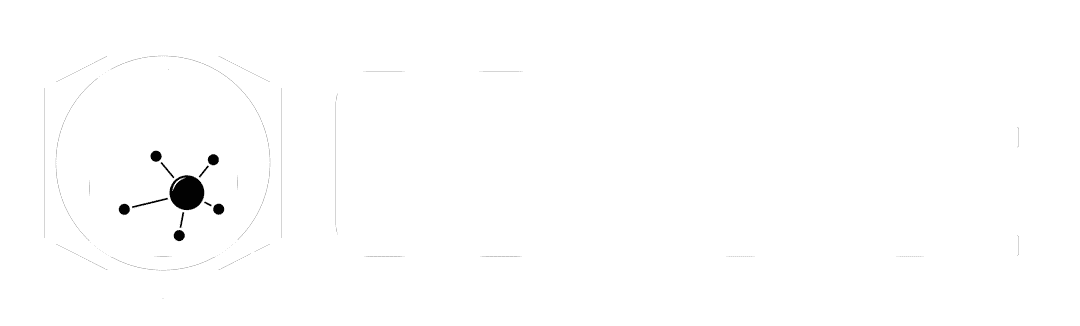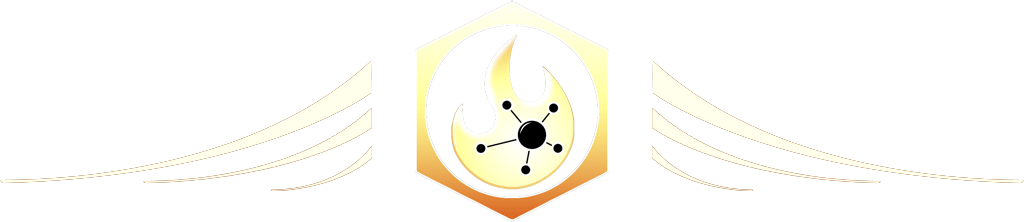# Match Size

The Ultimate Houdini node reference

# Visit the Node Bible

### Match Size

The Match Size node allows you to change the size, position, and alignment of an object based on the bounding box of another object.
Drag to resize

Summary:

According to the docs, "The Match Size operation moves and scales the first input to match the second input. If no second input is present, the provided position and size are used to define the destination bounding box."  In practice, you can use this to set an object perfectly on top of the scene grid, match the size of other objects, scale based on the bounding box of another object, or translate based on the location of another object.

Drag to resize

General Parameters:

Group:

--  This allows you to specify which polygons or points that you'd like to affect from the first input.

Justify With:

--  When the geometry in the first input goes to scale/transform/etc... it needs to look at a bounding box.  In the viewport, you can see this by the blue bounding box that has been drawn out.  This "Justify With" is asking you, "How would you like to create that blue bounding box?"

--  Input if Wired (default) =  It tries to find the bounding box by looking at the second input.  If it doesn't find any geometry, then it uses the bounding box found in the first geometry.

--  Origin and Unit Size =  Places the bounding box as a 1x1x1 cube at the origin

--  Second Input =  Looks at the second input.  If nothing is attached, then a bounding box will not be drawn and your geometry will disappear if scaled and placed at the origin.

--  Location and Size =  Defines a bounding box manually via the target position and size.

Use Groups to Justify Bounds:

--  This parameter allows you to select prims/points from the both the first and second input.  This may be confusing because we already have a "Group" parameter above.  This is different than the "Group" because we a selecting points/prims to figure out how things should move/translate around.  This is NOT which points/prims are allowed to be affected by this node.  The "Source Group" will look through the first input, find points/prims and use those points/prims bounds when scaling/translating the geo.  Points/Prims specified in the "Target Group" will be used when drawing out the bounding box around those selected prims/points.

Matching:

--  You can check on, "Translate," "Scale to fit," and "Uniform Scale" to toggle which operations get used on the first input geometry.

--  Justify X,Y,Z =  When translating, this node will look at the bounding box drawn around the first input and place it along the bounding box of the second input (if it is connected).  By default, Justify X,Y,Z is saying, "Take the center of the first input bounding box and place it at the center of the bounding box from the second input."  The option "Same" is poorly written in my opinion.  It's just saying, "If you use 'center' for the first bounding box, then use 'center' for the second input as well."  If you were to set it to "Max" and "Same," then the second geo would be set to "Max" because it's the 'same' as the first bounding box.  If you're not sure which setting you need, then just start picking an bunch of settings until you get what you're looking for.  Additional Offsets can be made through the offset sliders

-  Uniform Scale scales your object evenly when fitting inside the bounding box.

-  Scale Axis tells the node how it ought to scale the geo relative to the bounding box.

-  Best fit =  looks at the longest axis in x,y,z and scales it to fit by that.

-  Perimeter =  This parameter lacks sufficient documentation.  According to the docs, "The total edge lengths of the two models will be scaled to match. This is not well defined if either model lacks edges."  From my understanding, it takes a look at how long each edge is, sums it up, and then finds some kind of scaling ratio based on the length of those edges.  As an example, if you were to add a box to the second input and add more subdivisions, then it would increase the edge parameter value and scale up the model.

-  Area =  Similar to the "Perimeter," this setting is difficult to understand at first.  According to the docs, "The total surface area of the two models will be scaled to match. This is not well defined if either model has no surface."  So again, I imagine this as finding a ratio based on the surface area of polygons and using that to determine how it ought to be scaled.

-  Volume =  Same as Perimeter/Area except that you're measuring the volume of geo.

Restore & Stash Transform:

--  When transforming the object, you have the option to "Stash" the transform.  That will take an 8x8 transform matrix and save it as a detail attribute.  The idea here is that you can take this transform data and return it to the original location by inverting it.  As an example, you may want to take an object, scale it to the origin, do some work on it, and then put it back to where it originally was.  You can check on the, "Restore Transform" to apply the inverse transform and place the model back to where it originally came from.

Drag to resize